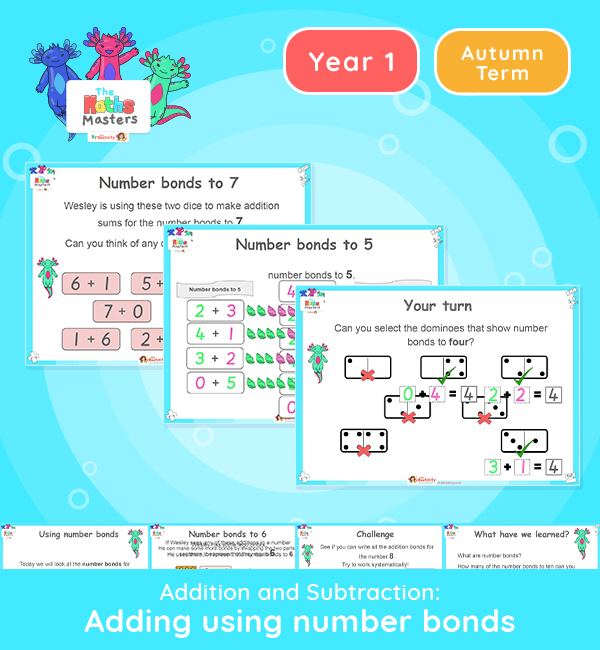# Year 1 | Adding Using Number Bonds Lesson Presentation## White Rose year 1 addition and subtraction resources

Aligned with the maths mastery approach, this Year 1 | Adding Using Number Bonds Lesson Presentation is fully editable and designed for the Year 1 maths curriculum to cover the following curriculum objectives:This lesson is a refresher lesson on number bonds for numbers up to 10 and how we use them when adding two numbers.

NC Links: Represent and use number bonds and related subtraction facts within 10. • Read, write and interpret mathematical statements involving addition (+), and equals (=) signs. • Add one digit numbers to 10, including zero. • Solve one step problems that involve addition using concrete objects and pictorial representations.

Previous Experience: Devise and record number stories, using pictures, numbers and symbols.

Future applications: Represent composition and decomposition of numbers using equations.

1AS-1  Compose numbers to 10 from 2 parts, and partition numbers to 10 into parts..

Future applications: Add and subtract within 10.

TAF Statements: Working Towards: *Add and subtract (one digit numbers) explaining their method verbally in pictures or using apparatus. * Recall at least four of the six number bonds for 10 and reason about associated facts

Working At: Recall all the number bonds to and within 10, and use these to reason with.

Aligned with the order of teaching of the maths mastery approach, use this to help your pupils get to grips with each mathematical concept. This lesson presentation also includes varied fluency activities, problem solving, and mathematical discussion questions too.

Explore our other year 1 addition and subtraction resources.

## Recently Viewed